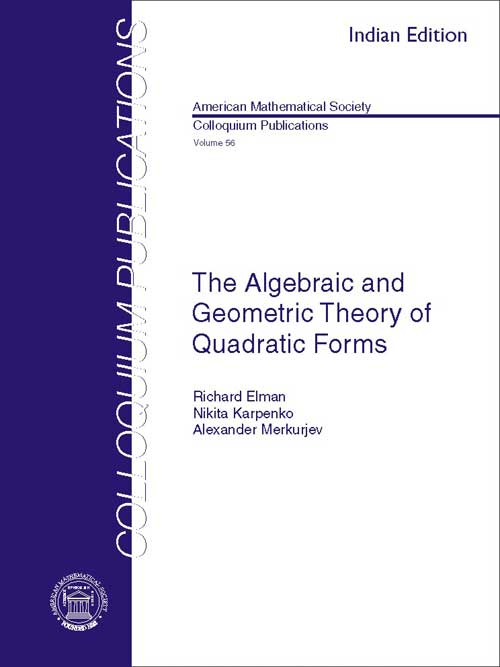The Algebraic and Geometric Theory of Quadratic Forms
Richard Elman, Nikita Karpenko, Alexander Merkurjev
Price
1845.00
ISBN
9780821868768
Language
English
Pages
448
Format
Paperback
Dimensions
180 x 240 mm
Year of Publishing
2011
Territorial Rights
Restricted
Imprint
American Mathematical Society
Catalogues

This book is a comprehensive study of the algebraic theory of quadratic forms, from classical theory to recent developments, including results and proofs that have never been published. The book is written from the viewpoint of algebraic geometry and includes the theory of quadratic forms over fields of characteristic two, with proofs that are characteristic independent whenever possible. For some results both classical and geometric proofs are given.

Part I includes classical algebraic theory of quadratic and bilinear forms and answers many questions that have been raised in the early stages of the development of the theory. Assuming only a basic course in algebraic geometry, Part II presents the necessary additional topics from algebraic geometry including the theory of Chow groups, Chow motives, and Steenrod operations. These topics are used in Part III to develop a modern geometric theory of quadratic forms.

Richard Elman is a professor of mathematics at University of California, Los Angeles, CA.
Nikita Karpenko is a professor of mathematics at Université Pierre et Marie Curie, Paris, France.
Alexander Merkurjev is a professor of mathematics at University of California, Los Angeles, CA.

Introduction

Part 1. Classical theory of symmetric bilinear forms and quadratic forms

Chapter I. Bilinear Forms
Chapter III. Forms over Rational Function Fields
Chapter IV. Function Fields of Quadrics
Chapter V. Bilinear and Quadratic Forms and Algebraic Extensions
Chapter VI. u-invariants
Chapter VII. Applications of the Milnor Conjecture
Chapter VIII. On the Norm Residue Homomorphism of Degree Two

Part 2. Algebraic cycles

Chapter IX. Homology and Cohomology
Chapter X. Chow Groups
Chapter XI. Steenrod Operations
Chapter XII. Category of Chow Motives
Part 3. Quadratic forms and algebraic cycles

Chapter XIII. Cycles on Powers of Quadrics
Chapter XIV. The Izhboldin Dimension
Chapter XV. Application of Steenrod Operations
Chapter XVI. The Variety of Maximal Totally Isotropic Subspaces

Appendices
Bibliography
Notation
Terminology
THE BOOKPOINT (INDIA) PVT. LTD.